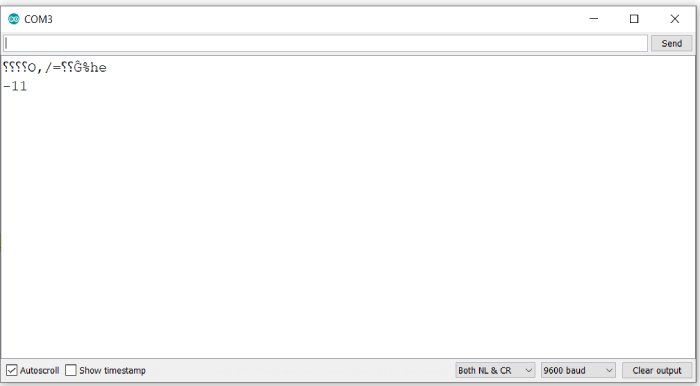# Bitwise NOT in Arduino

Unlike logical NOT, which inverts the truth value of an expression, the bitwise NOT applies to each bit of a number and inverts its value (0 to 1 and 1 to 0). The operator is ~.

The syntax thus is ~a, where a is the number on which this operator has to apply.

Please note, that all the leading 0s in the number’s representation are also converted to 1. For example, if your board uses 16 bits to represent an integer, then here’s what ~10 will look like

 0 0 0 0 0 0 0 0 0 0 0 0 1 0 1 0 10 1 1 1 1 1 1 1 1 1 1 1 1 0 1 0 1 ~10=-11

As you can see, each bit of 10 got inverted. This number corresponds to, using 2’s complement, -11. You can use this site for quickly converting decimal number to their 2’s complements and vice versa https://www.exploringbinary.com/twos-complement-converter/.

## Example

Let’s verify this on the Serial Monitor. The code is given below −

void setup() {
// put your setup code here, to run once:
Serial.begin(9600);
Serial.println();

int a = 10;
Serial.println(~a);
}

void loop() {
// put your main code here, to run repeatedly:
}

## Output

The Serial Monitor output is −As you can see, the output was exactly as expected.